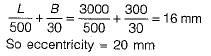Courses

# Columns - Civil Engineering

## 10 Questions MCQ Test Mock Test Series for Civil Engineering (CE) GATE 2020 | Columns - Civil Engineering

Description
This mock test of Columns - Civil Engineering for Civil Engineering (CE) helps you for every Civil Engineering (CE) entrance exam. This contains 10 Multiple Choice Questions for Civil Engineering (CE) Columns - Civil Engineering (mcq) to study with solutions a complete question bank. The solved questions answers in this Columns - Civil Engineering quiz give you a good mix of easy questions and tough questions. Civil Engineering (CE) students definitely take this Columns - Civil Engineering exercise for a better result in the exam. You can find other Columns - Civil Engineering extra questions, long questions & short questions for Civil Engineering (CE) on EduRev as well by searching above.
QUESTION: 1

### The effective length of a column in a reinforced concrete building frame, as per IS:456-2000, is independent of the

Solution:

Effective length of a column depends upon:
(i) Flexural stiffness (El/L) of beams joining at a point.
(ii) Flexural stiffness (El/L) of columns joining at a point.
(iii) Type of frame (sway or non-sway).

QUESTION: 2

Solution:
QUESTION: 3

### The reduction coefficient of a reinforced concrete column with an effective length of 4.8 m and size 250 mm x 300 mm is

Solution:

In the working stress method the design of a long column is made by considering reduced stresses obtained by multiplying the permissible stresses by a coefficient,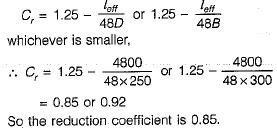QUESTION: 4

Which of the following statements is incorrect?

Solution:
QUESTION: 5

The ratio of the maximum and minimum percentage of longitudinal reinforcement that can be provided in a column is

Solution:

Maximum reinforcement = 6%
Minimum reinforcement = 0.8%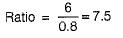QUESTION: 6

In a Pedestal, the factor by which the effective length should not exceed the least lateral dimension is

Solution:

Very short columns with effective length less than three times least lateral dimension are called pedestal columns.

QUESTION: 7

A reinforced concrete beam of 10 m Effective span and 1 m effective depth is supported on 500 mm x 500 mm columns. If the total uniformly distributed load on the beam is 10 MN/m, the design shear force for the beam is

Solution:

The shear force should be calculated at critical section i.e. 1 m away from the face of the column. Effective span of beam is to be taken as the clear span plus effective depth of beam or centre to centre spacing of supports which ever is less. The location for shear calculation will be,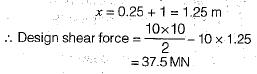QUESTION: 8

Which of the following are the additional moments considered for design of slender compression member in lieu of deflection in x and y directions?

where Pu is axial load; lex and ley are effective lengths in respective directions; D is depth of section perpendicular to major axis; b is width of the member)

Solution: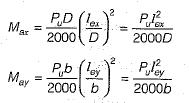QUESTION: 9

What is the minimum number of longitudinal bars provided in a reinforced concrete column of circular cross-section?

Solution:

As per IS : 456-2000 clause 26.5.3.1 (a) the minimum number of longitudinal bars provided in a column shall be four in rectangular columns and six in circular columns.

QUESTION: 10

An axially loaded column is of 300 mm x 300 mm size. Effective length of column is 3 m. What is the minimum eccentricity of the axial load for the column?

Solution:

Minimum eccentricity equals to unsupported length of column/500 plus lateral dimension/30, subject to a minimum of 20 mm.